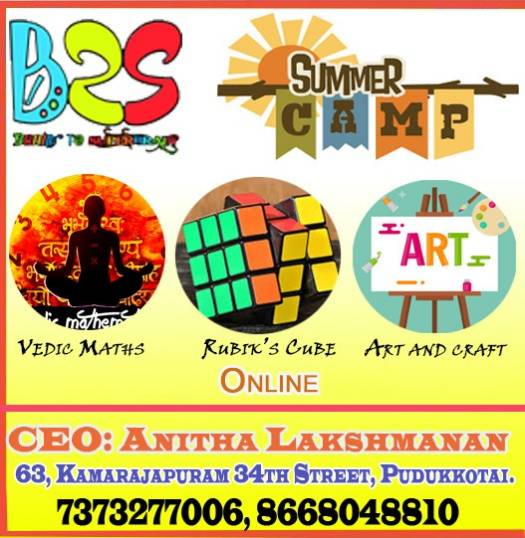Hi,

Are you or your children or your friends struggling with Math? Do you want to become a human calculator? If yes, then what are you waiting for? Enroll yourself in this course and have fun with Math. This course contains the world’s fastest Vedic maths techniques. It contains many solved examples, and exercises. This course contained examples that are explained in detail. how many of you can solve the questions in given time.

Can you find the Cube Root of 778688 in 5 seconds?

Can you Multiply 103 x 107 in 5 seconds?

Can you multiply 938643 x 999999 in 8 Seconds?

Can you write the tables from 1-100 without remembering?

Can you find cube and Square of any numbers from 1-100 in 8 seconds?

Can you multiply any two three digit numbers in 10 seconds?

Can you find the remainder of 9538792648 divided by 8 in 10 seconds?

Can you tell what is final price of product after 3 continuous Discounts by calculating in Mind?

Can you eliminate the Answers, if contains a complex calculations, by without solving them exactly?

If not then through this course you will learn high-speed Vedic mathematics, which will enable you to calculate much faster. You will be able to calculate difficult calculations in seconds which will boost your confidence Math is just calculation so this course will improve your calculation skill and you will be able to perform better.

• You would be learning how to speed up calculations which are blocks in mathematics.

• Sharpens your mind and increase’s intelligence in all your day to day activities.

• Improves memory and boost self-confidence

• Become a mental calculator for yourself

• Eradicates fear of math completely.Vedic Math, Rubik’s Cube, Arts Summer Camp 2021
Tagged on:
error: Content is protected !!